Courses

# First-Order & Second Order Reaction - Chemical Kinetics Chemistry Notes | EduRev

## Physical Chemistry

Created by: Asf Institute

## Chemistry : First-Order & Second Order Reaction - Chemical Kinetics Chemistry Notes | EduRev

The document First-Order & Second Order Reaction - Chemical Kinetics Chemistry Notes | EduRev is a part of the Chemistry Course Physical Chemistry.
All you need of Chemistry at this link: Chemistry

(2)  First-order reaction

Consider the following elementary reaction
A  → P
If the reaction is first order with respect to [A], the rate law expression is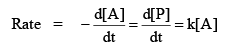k is rate constant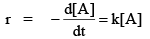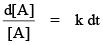If t = 0, the init ial concentration is [A]0 and the concentration at t = t, is [A], then integrating yields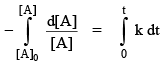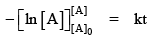[A] = [A]0 e–kt…(i)
or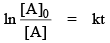…(ii)

Using this idea, the concentration of product with time for this first-order reaction is :

[P] + [A] = [A]0
[P] = [A]0 – [A]
[P] = [A]0 – [A]0 e–kt
[P] = [A]0 (1 – e–kt) ….(iii)

Graph representation of first order reaction

[A] = [A]0 e–kt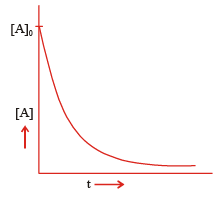Plot of concentration vs time.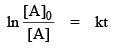ln [A] = –kt + ln [A]0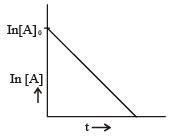Plot of log [A] vs time.

t1/2 i.e. half life t ime of first order reaction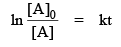when t = t1/2; then [A] =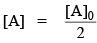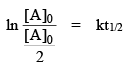ln 2 = kt1/2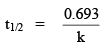Problem. The half life for the first order decomposition of N2O5 is 2.05 � 10s. How long will it take for a sample of N2O5 to decay to 60% of its initial value?

Sol. We know that,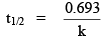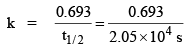The time at which the sample has decayed to 60% of its initial value then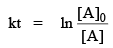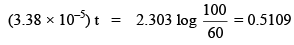T = 1.51 x 104 s
Problem. Find the t3/4 i.e. 3/4 life time of first order reaction.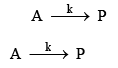Sol.

Integrated rate law expression iswhen t = t3/4 than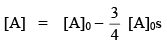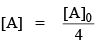then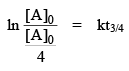ln 4 = kt3/4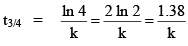(3)  Second-order reaction: (Type I)

Consider the following elementary reaction,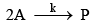If the reaction is second order with respect to [A], the rate law expression is rate =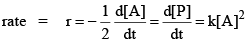k is rate constant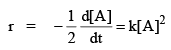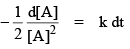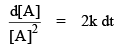If t = 0, the init ial concentration is [A]and the concentration at t = t, is [A], then integration yields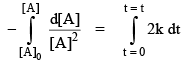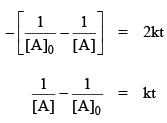…(i)

The concentration of product with time for second order reaction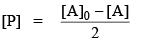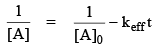or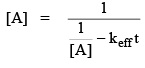then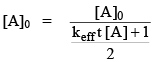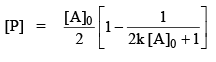…(ii)

t1/2 i.e. Half-life t ime of second order reaction (type I)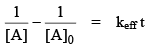when t = t1/2 then

⇒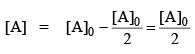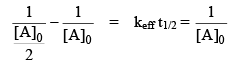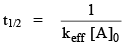Second-order reaction (Type II)

Second order reactions of t ype II invo lves two different reactants A and B, as fo llows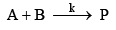Assuming that the reaction is first order in both A and B, the reaction rate is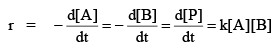If t = 0 then the init ial concentration are [A]0 & [B]and the concentration at t = t, are [A] & [B].

The loss of reactant i.e. the formation of product is equal to

[A]0 – [A] = [B]0 – [B] = [P]
[B]0 – [A]0 + [A] = [B]
then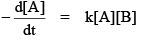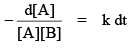the integration yield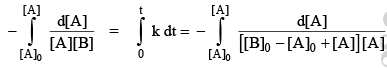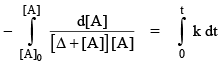let Δ = [B]0 – [A]
The solution to the integral involving [A] is given by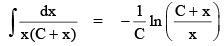Using this so lut ion to the integral, the integrated rate law expressio n beco mes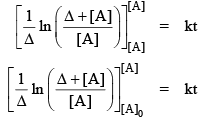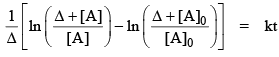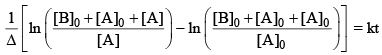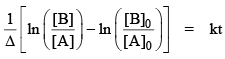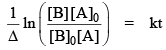⇒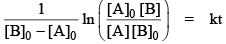Graph representation of second order reaction of type I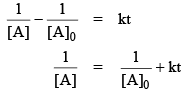Y = mx + C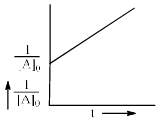Plot of concentration vs time

(4)  nth order reaction where n ≥ 2 :

An nth order reaction may be represented as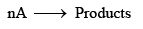the rate law is,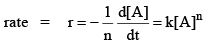where k is rate constant for nth order reaction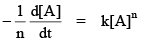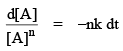If at t = 0, the init ial concentration is [A]0 and the concentration at t = t, is [A], then integration yields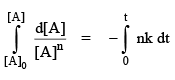Let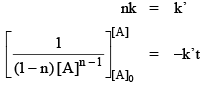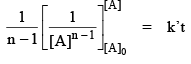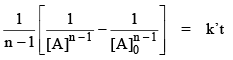…(1)
t1/2 i.e. Half life time of nth order reaction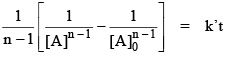Where t = t1/2 then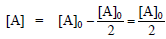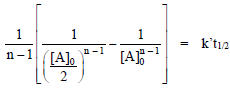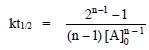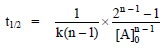…(2)

i.e.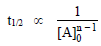…(3)

Thus we can say that t1/2 of the reaction is inversely proportional to the init ial concentration of reactant, except first order reaction.

So, for a first order reaction (n = 1), t1/2 is independent on [A]0 for a second order reaction (n = 2), t1/2 is dependent on [A]0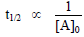for a nth order reaction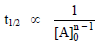Note : For the elementary reaction, the order of reaction is equal to the molecularity of the reaction.

Problem. Find the rate law for the following reaction.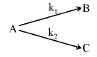Sol.Rate law is

Problem. Find the rate law for the following reaction.

Sol.

(1)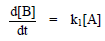(2)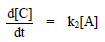(3)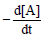= k1[A] + k2[A] = (k1 + k2)[A]

Problem. Find the rate law for the following reaction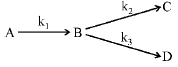Sol.

(1)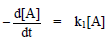(2)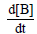= k1[A] – k2[B] – k3[B]
= k1[A] – (k2 + k3) [B]
(3)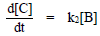(4)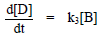Offer running on EduRev: Apply code STAYHOME200 to get INR 200 off on our premium plan EduRev Infinity!

## Physical Chemistry

77 videos|83 docs|32 tests

,

,

,

,

,

,

,

,

,

,

,

,

,

,

,

,

,

,

,

,

,

;